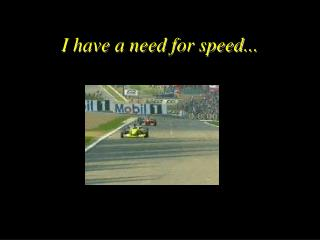DownloadDownload PresentationI have a need for speed...

# I have a need for speed...

Télécharger la présentation## I have a need for speed...

- - - - - - - - - - - - - - - - - - - - - - - - - - - E N D - - - - - - - - - - - - - - - - - - - - - - - - - - -
##### Presentation Transcript

1. I have a need for speed...

2. What is speed ?(km/h)

3. How do we calculate speed? • Gilles-Villeneuve Site of the Canadian Grand Prix. The speedway is 4.421 kms (2.747 miles) in length. The race length is 305.049 kms (189.548 miles) with 69 laps. The course record was set in 1998 by Michael Schumacher in a Ferrari F310B V10 with a time of 1 min 19.379 sec and a speed of 200.45km/h (124.581 miles/h). • Monza Site of the Italian Grand Prix. The speedway is 5.77 kms (3.585 miles) in length. The race length is 305.548 kms (189.858 miles) with 53 laps. The course record was set in 1997 by Mr. Hakkinen in a McLaren MP4 / V10 with a time of 1 min 24.808 sec and a speed of 244.929km/h (152.192 miles/h). The speedway is the fastest on the agenda, with average speeds of 250 km/h and speeds of more than 350 km/h are the norm.

4. Speed = distance ÷ timev = d ÷ t Speed : describes how quickly an object is moving. It is a scalar quantity, meaning it does not indicate a direction. Example 1 : at Monza, speeds greater than 350km/hr are the norm. Exemple 2 : High-speed elevators (like the ones in the CN Tower in Toronto), can attain speeds of 7.11m/s. How much time would it take to go 37.5 m (about 10 floors) ?

5. Average speed versus instantaneous speed… When an object moves, it undergoes frequent changes in speed. For example, in an average trip to school, there are many changes in speed. The needle in your car is constantly moving up and down to reflect the stopping, starting and acceleration. At one point you could be going 50 km/hr and at another point, you could be stopped (that is to say, 0 km/hr). However, during the course of your drive, your average speed may only be 25 km/hr. Average speed can be calculated by the following equation: Average speed = Distance travelled time

6. On vacation, Lisa Carr went a total distance of 440 km. Her run took 5 hours. What was her average speed? vm = d / t vm= 440km / 5 hr vm= 88km/hr Lisa Carr went an average speed of 88 km per hour. She couldn’t have gone at a constant speed of 88 km/hr. Without a doubt, she stopped (0 km/hr) at some point (possibly for a bathroom break or to eat) and she most likely went 110 km/hr at other times. Therefore, on average she drove 88 km/h. Instantaneous speed – the speed of an object at a given moment in time. Average speed – the average of all the instantaneous speeds; found simply by the ratio of distance/time.

7. Draw the following distance – time graph.

8. Questions about your graph: 1. What does the line represent ? 2. Calculate the average speed of the object. 3. Calculate the speed at t = 4s. 4. Draw two more lines on your graph: one which represents an object going a slower speed and the other which represents an object going a faster speed.

9. What is the difference between speed and velocity ? Speed = Distance / Time Velocity = Displacement / Time

10. Speed Scalar value dependent on the distance Accompanied by a unit representing distance over time Ex: He drove the bus at 60 km/h Velocity Vector value dependent on the displacement, indicating the direction of the displacement Ex: The bird flies at 72 km/h to the North Speed vs. Velocity